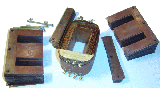# Size of External Iron

We've looked at the general iron shape available from surplus transformer cores. Now we wonder what size of core to choose, and how to figure out how much is enough.

## Size of External IronWhen choosing a surplus transformer the question is: What width ‘w’ of the core leaf is “big enough”? Or, what size of core results in “extra material” and becomes wasted mass?

One approach is to assume a massive simplification of the magnetic circuit, solvable by Ohm’s Law if we neglect fringing and leakage. This approach will treat the magnetic path as a collection of series resistors, representing the nail and air gap and iron core material.

We want a “small” core resistance (reluctance), of 10% or less of the other resistance:

Rcore < 10% of (Rnail + Rair gap)

Let’s assume Rair is negligible (it’s not because permeability of air TR = 1) for the sake of finding a limit on Rcore. This is again a gross simplification, but it errs on the side of suggesting an iron core width ‘w’ larger than necessary.

Since the Rnail is limited by its cross-section, all we need is the transformer core cross-section to be 10x or more than the nail’s.

If the nail’s diameter is 2.8mm (our largest projectile), then:

Anail = Πr2 = 6.157 x 10-6 meters sq.

Since our physical core assembly is 10mm thick, the core’s cross-section:

Acore = width * height = (10mm) * w

Minimum ‘w’ = (10)*(Acore)/(10 mm) = 6 mm

Since our physical core was built with w = 17mm, we conclude it has extra mass and could be made smaller. For now, we’ll leave it as-is and take advantage of its lower magnetic reluctance.

 < Previous Page 15 of 37 Next >